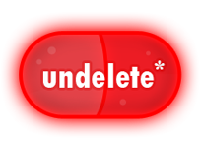### UndeletePillUsed by

This undelete "pill" needs to reach the bottom in the level "Deliverance", to trigger the end of the level.

```<ball name="UndeletePill"
shape="circle,80"
mass="30"
towermass="30"
strands="0"
walkspeed="0"
climbspeed="0"
speedvariance="0"
collidewithattached="true"
suckable="false"
jump="0.0,0.0"
climber="false"
draggable="false"
material="rock"
contains="80,UndeletePillFizz"
popsound="SOUND_BALL_UNDELETEPILL_POP"
popdelay="2,2"
popduration="1.0"
popparticles="beautypop"
isbehindstrands="true"
wakedist="600"
invulnerable="true"

autoboundsunattached="true"

dampening="0.1"
>

<part name="body"
layer="0"
rotate="true"
x="0" y="0"
image="IMAGE_BALL_UNDELETEPILL_BODY"
stretch="18,1.25,0.9"
scale="1.0"
/>

</ball>```